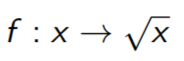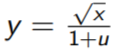# 用R进行模拟

```> x

plot(table(x), main = "frequency")```

2. 数字变量 按照高斯分布N（μ=1，σ=1）随机生成一个大小为100的向量。

`> x hist(x, main = "")`

# 模拟数据1. 定义输入的矢量为x∼U[0,1]

2. 定义一个向量u∼N+(µ = 0.25, σ = 0.2)

3. 输入的矢量定义为```> u = rtmvnorm(n = ns, mean = c(0.25), sigma = c(0.2),
+ lower = c(0))
> y = ybar/(1 + u)```

# 数据的表示方法

`> plot(y ~ x`

`> lines(t.fr ~ x.seq)`

# “真实前沿 “效率测算

Shepard 方法:

```> lambda = y/sqrt(x)
> theta = y^2/x
> delta = 1/theta```

# 可重复研究

```> matable <- xtable(tab1\[1:5, \], digits = 3, align = "l|ccc",
+ caption = ""真实前沿效率测算")```

# 随机前沿

1.用函数lm调整一个线性模型，并保留回归线的系数β：y = α + βx

2. 找到能使（yi-yˆi）最大化的公司k，i=1，…，n。注意，公司k可以通过函数识别来手动找到和检测

3. 计算α 0，使回归线y = α 0 + βx穿过企业k，并代表随机前沿。

## 1. OLS 模型

`> res.lm <- lm(y ~ x)`

# R语言时变面板平滑转换回归模型TV-PSTR分析债务水平对投资的影响

## 2. 使用函数识别

`> identify(x, y)`

## 3. 找到α0

```> alpha2 <- y\[3\] - beta.lm\[2\] *
+ x\[3\]
> plot(y ~ x, type = "p",
+ 1))```

# 随机前沿效率测算

`> f1 = function(x) alpha2 + beta.lm\[2\] * x`
`> f1.inv = function(x) (x - alpha2)/beta.lm\[2\]`

Shepard 方法:

# DEA – FDH 表示

```> plot(y ~ x)
> lines(x\[c(2, 9, 3, 4)\], legend = c("DEA",
+ "FDH"))```

# Naive Bootstrap

1.用函数样本在15个观测值中取样

2.计算前沿的新估计值

3.计算新的效率方法

4. 储存结果，计算偏差, 方差, 置信区间

# 对真实数据的分析

## 数据集

```> plot(y ~ x1,pch = 16, col = "blue")
> abline(lm(y ~ x1, data), col = "red")```

# 散点图3-d

```> scatterplot3d(x1,
+ x2, y)```

# 数据结构

p个输入包含在一个p×n的矩阵中

`> input <- t(cbind(spain\$x1, spain\$x2, spain\$x3))`

q个输出包含在一个q×n的矩阵中

`> output <- t(matrix(spain\$y))`

# 效率的衡量标准

```> res <- rbind(dea, fdh, orderm,
+ res.hquan)```

`> plot(density(res.dea)`

# Bootstrap

`> boot(input, output)`

Kaizong Ye拓端研究室（TRL）的研究员。

​非常感谢您阅读本文，如需帮助请联系我们！QQ在线咨询

15121130882

0571-63341498

## 关注有关新文章的微信公众号

This will close in 0 seconds